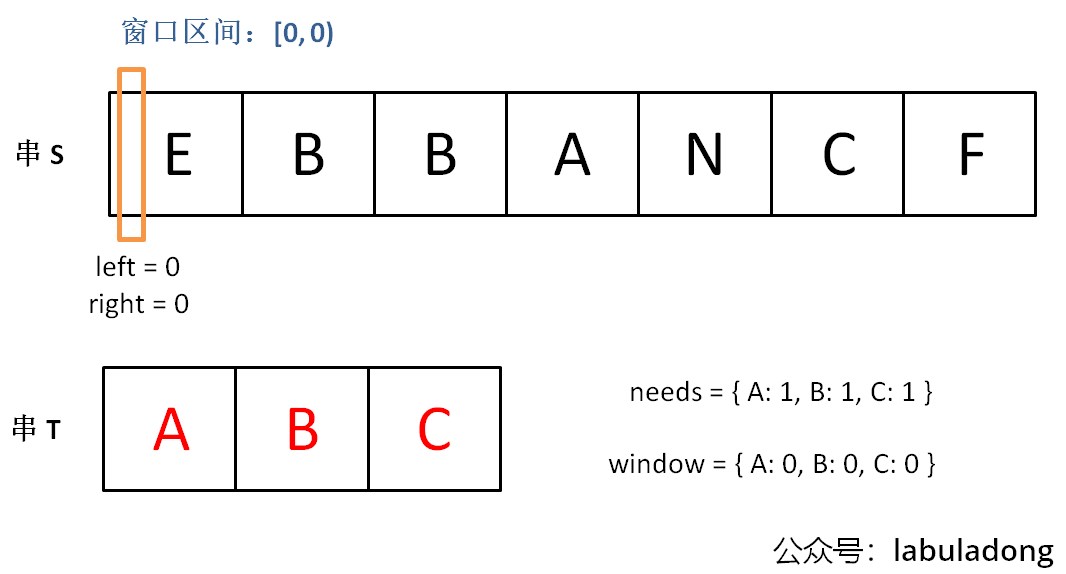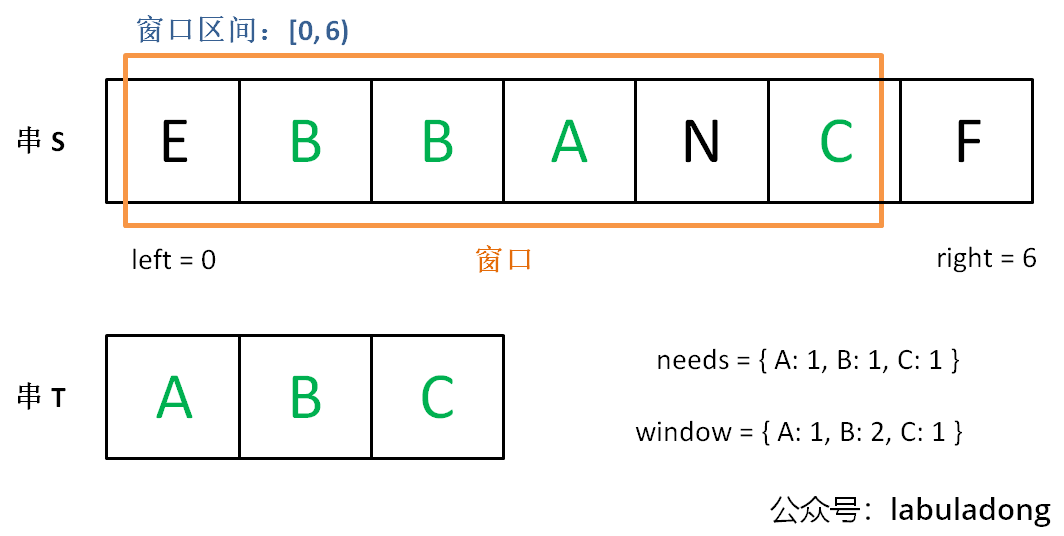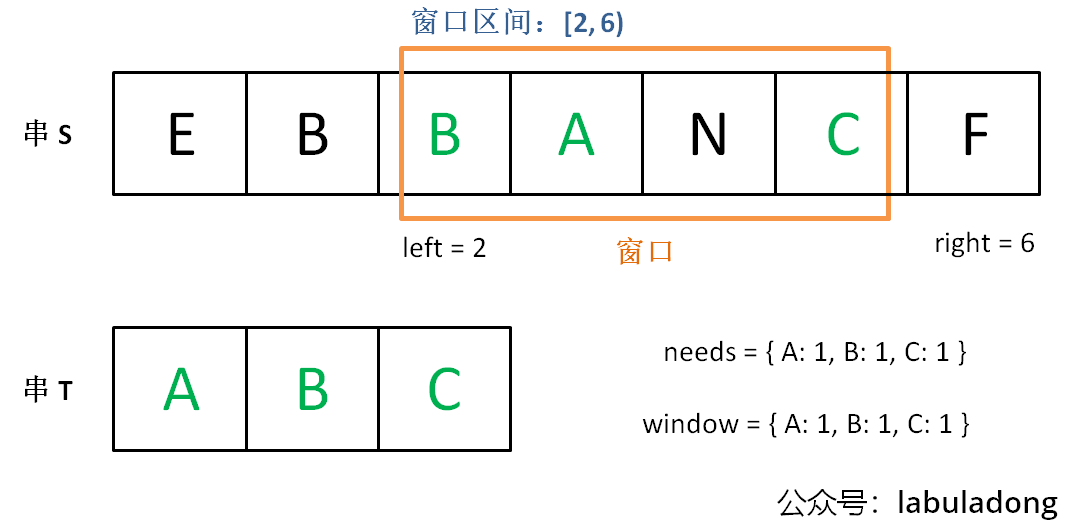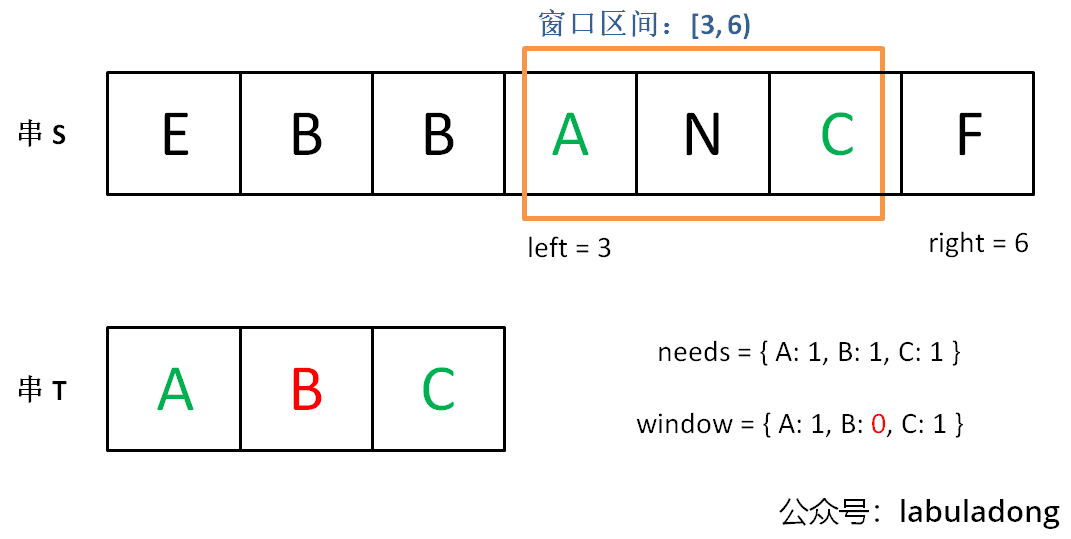Info

Tip``````int left = 0, right = 0;

while (left < right && right < s.size()) {
// 增大窗口
right++;

while (window needs shrink) {
// 缩小窗口
window.remove(s[left]);
left++;
}
}
``````

``````/* 滑动窗口算法框架 */
void slidingWindow(string s) {
// 用合适的数据结构记录窗口中的数据
unordered_map<char, int> window;

int left = 0, right = 0;
while (right < s.size()) {
// c 是将移入窗口的字符
char c = s[right];
// 增大窗口
right++;
// 进行窗口内数据的一系列更新
...

/*** debug 输出的位置 ***/
// 注意在最终的解法代码中不要 print
// 因为 IO 操作很耗时，可能导致超时
printf("window: [%d, %d)\n", left, right);
/********************/

// 判断左侧窗口是否要收缩
while (left < right && window needs shrink) {
// d 是将移出窗口的字符
char d = s[left];
window.remove(d)
// 缩小窗口
left++;
// 进行窗口内数据的一系列更新
...
}
}
}
``````

`unordered_map` 就是哈希表（字典），相当于 Java 的 `HashMap`，它的一个方法 `count(key)` 相当于 Java 的 `containsKey(key)` 可以判断键 key 是否存在。

### # 一、最小覆盖子串``````for (int i = 0; i < s.size(); i++)
for (int j = i + 1; j < s.size(); j++)
if s[i:j] 包含 t 的所有字母:
更新答案
``````

1、我们在字符串 `S` 中使用双指针中的左右指针技巧，初始化 `left = right = 0`，把索引左闭右开区间 `[left, right)` 称为一个「窗口」。

Tip

2、我们先不断地增加 `right` 指针扩大窗口 `[left, right)`，直到窗口中的字符串符合要求（包含了 `T` 中的所有字符）。

3、此时，我们停止增加 `right`，转而不断增加 `left` 指针缩小窗口 `[left, right)`，直到窗口中的字符串不再符合要求（不包含 `T` 中的所有字符了）。同时，每次增加 `left`，我们都要更新一轮结果。

4、重复第 2 和第 3 步，直到 `right` 到达字符串 `S` 的尽头。``````unordered_map<char, int> need, window;
for (char c : t) need[c]++;
``````

``````int left = 0, right = 0;
int valid = 0;
while (right < s.size()) {
// 开始滑动
}
``````

1、什么时候应该移动 `right` 扩大窗口？窗口加入字符时，应该更新哪些数据？

2、什么时候窗口应该暂停扩大，开始移动 `left` 缩小窗口？从窗口移出字符时，应该更新哪些数据？

3、我们要的结果应该在扩大窗口时还是缩小窗口时进行更新？

``````string minWindow(string s, string t) {
unordered_map<char, int> need, window;
for (char c : t) need[c]++;

int left = 0, right = 0;/**<extend down -200><div class="img-content"><img src="/algo/images/slidingwindow/1.png" class="myimage"/></div> */
int valid = 0;
// 记录最小覆盖子串的起始索引及长度
int start = 0, len = INT_MAX;
while (right < s.size()) {
// c 是将移入窗口的字符
char c = s[right];
// 扩大窗口
right++;
// 进行窗口内数据的一系列更新
if (need.count(c)) {
window[c]++;
if (window[c] == need[c])
valid++;
}

// 判断左侧窗口是否要收缩
while (valid == need.size()) {/**<extend down -200><div class="img-content"><img src="/algo/images/slidingwindow/2.png" class="myimage"/></div> */
// 在这里更新最小覆盖子串
if (right - left < len) {
start = left;
len = right - left;
}
// d 是将移出窗口的字符
char d = s[left];
// 缩小窗口
left++;
// 进行窗口内数据的一系列更新
if (need.count(d)) {
if (window[d] == need[d])
valid--;
window[d]--;
}
}/**<extend up -50><div class="img-content"><img src="/algo/images/slidingwindow/4.png" class="myimage"/></div> */
}
// 返回最小覆盖子串
return len == INT_MAX ?
"" : s.substr(start, len);
}
``````

Warning

`valid == need.size()` 时，说明 `T` 中所有字符已经被覆盖，已经得到一个可行的覆盖子串，现在应该开始收缩窗口了，以便得到「最小覆盖子串」。

### # 二、字符串排列``````// 判断 s 中是否存在 t 的排列
bool checkInclusion(string t, string s) {
unordered_map<char, int> need, window;
for (char c : t) need[c]++;

int left = 0, right = 0;
int valid = 0;
while (right < s.size()) {
char c = s[right];
right++;
// 进行窗口内数据的一系列更新
if (need.count(c)) {
window[c]++;
if (window[c] == need[c])
valid++;
}

// 判断左侧窗口是否要收缩
while (right - left >= t.size()) {
// 在这里判断是否找到了合法的子串
if (valid == need.size())
return true;
char d = s[left];
left++;
// 进行窗口内数据的一系列更新
if (need.count(d)) {
if (window[d] == need[d])
valid--;
window[d]--;
}
}
}
// 未找到符合条件的子串
return false;
}
``````

1、本题移动 `left` 缩小窗口的时机是窗口大小大于 `t.size()` 时，因为排列嘛，显然长度应该是一样的。

2、当发现 `valid == need.size()` 时，就说明窗口中就是一个合法的排列，所以立即返回 `true`

Note

### # 三、找所有字母异位词``````vector<int> findAnagrams(string s, string t) {
unordered_map<char, int> need, window;
for (char c : t) need[c]++;

int left = 0, right = 0;
int valid = 0;
vector<int> res; // 记录结果
while (right < s.size()) {
char c = s[right];
right++;
// 进行窗口内数据的一系列更新
if (need.count(c)) {
window[c]++;
if (window[c] == need[c])
valid++;
}
// 判断左侧窗口是否要收缩
while (right - left >= t.size()) {
// 当窗口符合条件时，把起始索引加入 res
if (valid == need.size())
res.push_back(left);
char d = s[left];
left++;
// 进行窗口内数据的一系列更新
if (need.count(d)) {
if (window[d] == need[d])
valid--;
window[d]--;
}
}
}
return res;
}
``````

### # 四、最长无重复子串``````int lengthOfLongestSubstring(string s) {
unordered_map<char, int> window;

int left = 0, right = 0;
int res = 0; // 记录结果
while (right < s.size()) {
char c = s[right];
right++;
// 进行窗口内数据的一系列更新
window[c]++;
// 判断左侧窗口是否要收缩
while (window[c] > 1) {
char d = s[left];
left++;
// 进行窗口内数据的一系列更新
window[d]--;
}
// 在这里更新答案
res = max(res, right - left);
}
return res;
}
``````

`window[c]` 值大于 1 时，说明窗口中存在重复字符，不符合条件，就该移动 `left` 缩小窗口了嘛。

1、什么时候应该扩大窗口？

2、什么时候应该缩小窗口？

3、什么时候应该更新答案？